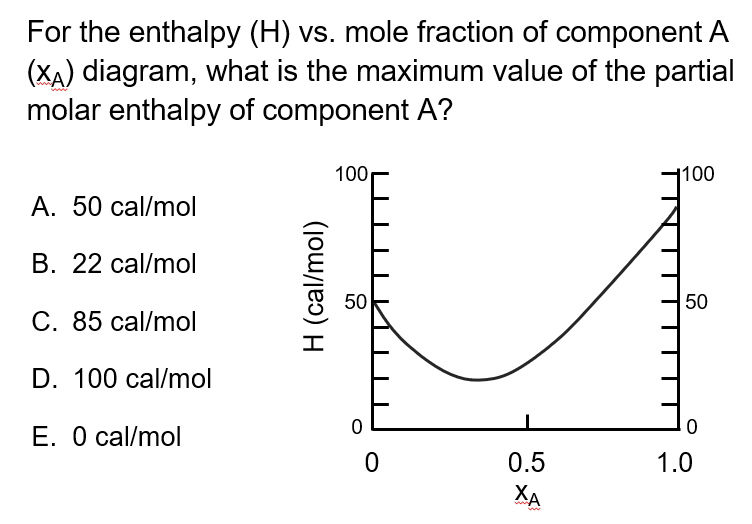LearnChemE

#### Partial Molar Quantities: ConcepTest and Example Problem

Try to answer this ConcepTest and solve the example problem before using this module. Studies show that trying to answer the questions before studying material improves learning and retention. We suggest that you write down the reasons for your answers. By the end of this module, you should be able to answer these on your own. Answers will be given at the end of this module.##### Example Problem 1

Determine a partial molar quantity given a molar quantity dependence on composition of a binary solution. Given that a molar quantity M equals 6x1x2 where x1 is the mole fraction of component 1 and x2 is the mole fraction of component 2, calculate the partial molar quantity of component 1.